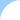Home Cooking Utilities Recipes Site IndexMetric Weights & Measures

 COOKING UTILITIES Spice RackMETRIC UNITS AND THEIR EQUIVALENTS

The metric system of measurement is a very organized system which is based on multiples of ten. For cooking, the three basic metric units are the liter, gram, and meter. All other units are derived from these by adding a prefix to the basic unit.

 PREFIX FRACTIONAL VALUE DECIMAL VALUE kilo 1000 1000 hecto 100 100 deca 10 10 deci 1/10 0.1 centi 1/100 0.01 milli 1/1000 0.001

To find the value of the unit, just multiply the value of the prefix by the base unit.
EXAMPLES:

1 kilogram = kilo x 1 gram = 1000 grams

1 centimeter = centi x 1 meter = 1/100th of a meter or 0.01 meters

3 milliliters = milli x 3 liters = 1/1000 x 3 liters = 3/1000 liters or 0.003 liters

VOLUME MEASUREMENTS

 milliliter centiliter deciliter liter decaliter hectoliter 1 milliliter = 1 0.1 0.01 0.001 0.0001 0.00001 1 centiliter = 10 1 0.1 0.01 0.001 0.0001 1 deciliter = 100 10 1 0.1 0.01 0.001 1 liter = 1000 100 10 1 0.1 0.01 1 decaliter = 10000 1000 100 10 1 0.1 1 hectoliter = 100000 10000 1000 100 10 1

WEIGHT

 milligram centigram decigram gram decagram hectogram kilogram 1 milligram = 1 0.1 0.01 0.001 0.0001 0.00001 0.000001 1 centigram = 10 1 0.1 0.01 0.001 0.0001 0.00001 1 decigram = 100 10 1 0.1 0.01 0.001 0.0001 1 gram = 1000 100 10 1 0.1 0.01 0.001 1 decagram = 10000 1000 100 10 1 0.1 0.01 1 hectogram = 100000 10000 1000 100 10 1 0.1 1 kilogram = 1000000 100000 10000 1000 100 10 1

LENGTH

 millimeter centimeter decimeter meter decameter hectometer kilometer 1 millimeter = 1 0.1 0.01 0.001 0.0001 0.00001 0.000001 1 centimeter = 10 1 0.1 0.01 0.001 0.0001 0.00001 1 decimeter = 100 10 1 0.1 0.01 0.001 0.0001 1 meter = 1000 100 10 1 0.1 0.01 0.001 1 decameter = 10000 1000 100 10 1 0.1 0.01 1 hectometer = 100000 10000 1000 100 10 1 0.1 1 kilometer = 1000000 100000 10000 1000 100 10 1